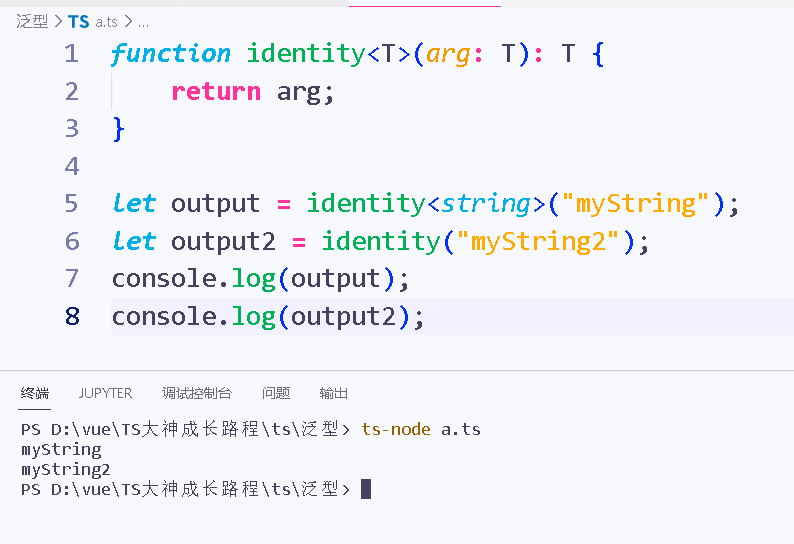﻿ TypeScript泛型的使用详细介绍_javascript技巧_脚本之家
javascript技巧# TypeScript泛型的使用详细介绍

## 情景再现

```function identity(arg: number): number {
return arg;
}
```

```function identity2(arg: string): string{
return arg;
}
```

```function identity(arg: any): any {
return arg;
}
```

```function identity<T>(arg: T): T {
return arg;
}
```

🐱‍🏍我们把这个版本的identity函数叫做泛型，因为它可以适用于多个类型。 不同于使用any，它不会丢失信息，像第一个例子那像保持准确性，传入数值类型并返回数值类型。

## 使用泛型```function identity<T>(arg: T): T {
return arg;
}
let output = identity<string>("myString");
let output2 = identity("myString2");
console.log(output);
console.log(output2);
```## 泛型类型

```function identity<T>(arg: T): T {
return arg;
}
let myFunction: <T>(arg:T) => T = identity;
```

```function identity<T>(arg: T): T {
return arg;
}
let myFunction: <T>(arg:T) => T = identity;
let myIdentity: <U>(arg: U) => U = identity;
```

```function identity<T>(arg: T): T {
return arg;
}
let myIdentity: {<T>(arg: T): T} = identity;
```

## 泛型接口

```interface GenericIdentityFn {
<T>(arg: T): T;
}
function identity<T>(arg: T): T {
return arg;
}
let myIdentity: GenericIdentityFn = identity;```

```interface GenericIdentityFn<T> {
(arg: T): T;
}
function identity<T>(arg: T): T {
return arg;
}
let myIdentity: GenericIdentityFn<number> = identity;```

## 泛型类

```// 泛型类
class GenericNumber<T> {
zeroValue: T | undefined;
add: ((x: T, y: T) => T) | undefined;
}
let myGenericNumber = new GenericNumber<number>();
myGenericNumber.zeroValue = 0;
return x + y;
};
return x + y;
};```// 泛型类
class GenericNumber<T> {
zeroValue: T | undefined;
add: ((x: T, y: T) => T) | undefined;
}
let stringNumeric = new GenericNumber<string>();
stringNumeric.zeroValue = "hi,";
stringNumeric.add = function(x, y) { return x + y; };## 泛型约束

```function loggingIdentity<T>(arg: T): T {
console.log(arg.length);  // Error: T doesn't have .length
return arg;
}
```

```function loggingIdentity<T>(arg: T[]): T[] {
console.log(arg.length);  // Array has a .length, so no more error
return arg;
}
```

```interface Lengthwise {
length: number;
}
function loggingIdentity<T extends Lengthwise>(arg: T): T {
console.log(arg.length);  // Now we know it has a .length property, so no more error
return arg;
}```## 在泛型里使用类类型[]

```function create<T>(c: {new(): T; }): T {
return new c();
}
```

### 高级案例

```class BeeKeeper {
}
class ZooKeeper {
nametag:string =  "ZooKeeper.nametag";
}
class Animal {
numLegs: number = 100;
}
class Bee extends Animal {
keeper: BeeKeeper = new BeeKeeper();
}
class Lion extends Animal {
keeper: ZooKeeper = new ZooKeeper();
}
function createInstance<A extends Animal>(c: new () => A): A {
return new c();
}
console.log(createInstance(Lion).keeper.nametag);